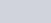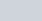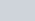# Convert candela to lumens using this calculator – I (cd) to ϕ (lm)

Lumens and candela are units of measurement for lighting, but they measure different aspects of a lighting fixture.

Lumens refers to the total amount of light that a lighting fixture emits, while candela refers to the amount of light emitted by a lighting fixture in a particular direction.

In this article you will find the calculator, formulas, examples, explanation and steps to easily convert from candela to lumens.

Mas información:

## Candela to lumen formula

To convert from candela to lumens, the value of candela must be multiplied by the angular interval of the light source in steradians, as shown in the following formula (1):Where, is the symbol of the lumens to find and, is the symbol of the candelas, while, it is the symbol of the steradians that are defined by the following formula (2):When making the replacement of the formula of the steradians (2) in the formula of candela to lumen (1), we have the following:In other words, the formula to convert from candela to lumen is as follows:## Steps to convert from candela to lumenStep 1: Obtain the candela and the vertex angle in degrees of the light source. (These values ​​are in catalogs and the internet).

Step 2: Enter the candela and degrees into the formula: lumens = candela × (2π (1 – cos (degrees / 2))) .

Step 3: Example: An LED luminaire produces 1.5 candela at a corner angle of 70 °, how many lumens does it produce? All you have to do is replace 1.5 cd and 70 ° C in the conversion formula, which will give you: 1.7lm.

## Candela and lumens definitions

Lumens and candela are units of measurement for lighting, but they measure different aspects of a lighting fixture.

Knowing the difference between lumens and candela will help you choose lighting fixtures that suit the needs of your workplace

More importantly, this knowledge will ensure that your lighting supplier does not mislead you, as they may try to convince you of the advantages of a lighting fixture by citing impressive lumens or candela numbers that are not relevant to your needs.

### Definition of candela

The candela (cd unit) has its origin in the brightness of a “standard candle”, but has received a more precise definition in the International System of Units (SI), and at that time the unit was also called a “candle” or “candle”.

The candela measures the amount of light emitted in the range of an angular (three-dimensional) span. Since light intensity is described in terms of an angle, the distance at which you measure this intensity is irrelevant.To facilitate illustration, in the image above the three dimensions have been reduced to two. In this image, screen B would capture exactly the same amount of light rays (emitted from the light source) as screen A, provided that screen A was removed so as not to obscure screen B. This is because the screen B covers the same angle as screen A.

The angular span of the candela is expressed in steradian, a unitless measure (this is like radians for angles in two-dimensional space, but they are not the same). A steradian on a sphere with a radius of one meter gives a surface area of ​​one m 2 . A complete sphere measures stereoradians.### Definition of lumen

If you look at LEDs, especially high-brightness LEDs, you may notice that they have a high luminous intensity (in candela or milli-candela, mcd) and usually have a narrow corner angle. Similarly, LEDs with a wide vertex angle tend to have a relatively low light intensity. The same applies to halogen spotlights with a reflector: those with a narrow-beam reflector have a higher candlepower than wide-beam spotlights with the same power.

The cause of this relationship is the total energy produced by the LED. LEDs of a specific class (eg “high flux”) all produce roughly the same amount of light energy. However, when an LED emits its full energy in a beam with a narrow angle, the intensity will be greater (in the direction of that angle) than when the same energy was emitted at a wide angle.

The lumen (unit lm) gives the total luminous flux of a light source by multiplying the intensity (in candela) by the angular range over which the light is emitted.

As a frame of reference, a standard 120V / 60W bulb has a wattage of 850 lm, and the equivalent 230V / 60W bulb has a wattage of 700 lm. A 20W low voltage (12V) tungsten halogen lamp produces approximately 310 lm.

### Definition of apex angle or vertex angle.

Since lumen and candela measurements are related through viewing angle (or vertex angle), it is helpful to know how this angle is defined.One measures the angle between the axis where the light source gives its greatest light intensity and the axis where that intensity is reduced to 50%. In the image above, this angle is denoted by θ. The angle of the vertex is twice that angle (which means = 2θ).

Note that the 50% dimming is based on a linear scale, but our perception of brightness is not linear. The CIE has standardized the relationship between light intensity and brightness perceived as a cube root; other sources claim that a square root best approximates this relationship.

The three-dimensional angular span for a vertex angle, using, for the angular span (in steradian) and 2θ for the vertex angle, is:## Examples of how to convert from candela to lumen

Example 1:  An LED for a motorcycle has 1114 candela with a vertex angle of 30 °, how many lumens does this LED lamp generate?

Rta: // Having the candela and the vertex angle, all we have to do is replace these values ​​in the conversion formula: lumens = candela × (2π (1 – cos (degrees / 2))), which will give us as result: 238 lm.

Example 2:  There is a led luminaire with a luminous flux in candela of 250 and a vertex angle of 60 °, how many lumens will the luminaire have?

Rta: // With the values ​​of the candela and the vertex angle it must be replaced in the conversion formula: lumens = candela × (2π (1 – cos (degrees / 2))), which will give us as a result: 210 lm.